# RD Sharma Solutions for Class 6 Maths Chapter 20: Mensuration Exercise 20.3

In previous exercises, students learn about perimeter, length and other parameters which are important from the exam point of view. This exercise mainly focuses on concepts like area and its measurement. The magnitude of the measurement of the region which is enclosed by a closed plane figure is known as its area. The problems in this exercise help students understand the measurement of area in various structures. They can refer to RD Sharma Solutions Class 6 Maths Chapter 20 Mensuration Exercise 20.3 PDF, which are available here, in order to perform well in the exam.

## RD Sharma Solutions for Class 6 Maths Chapter 20: Mensuration Exercise 20.3 Download PDF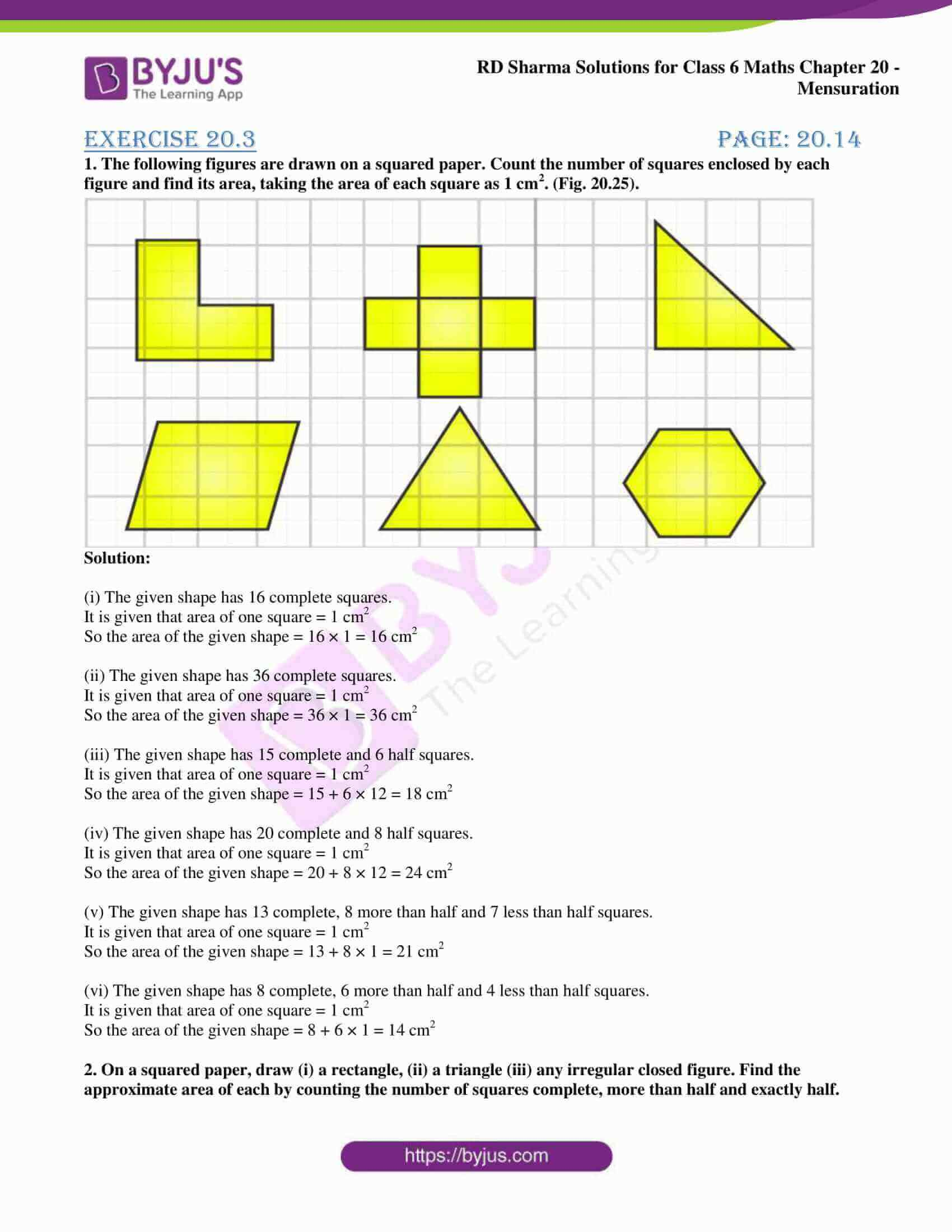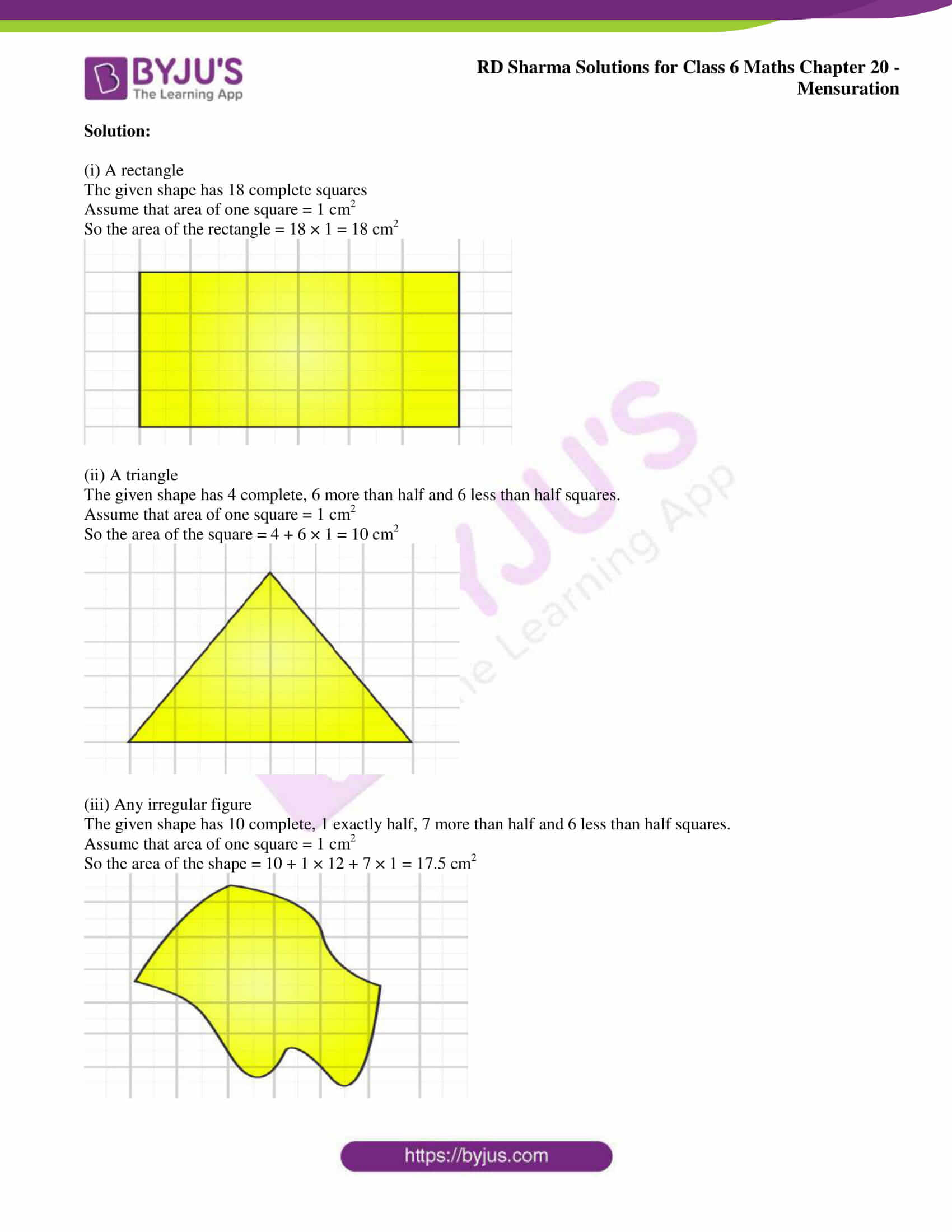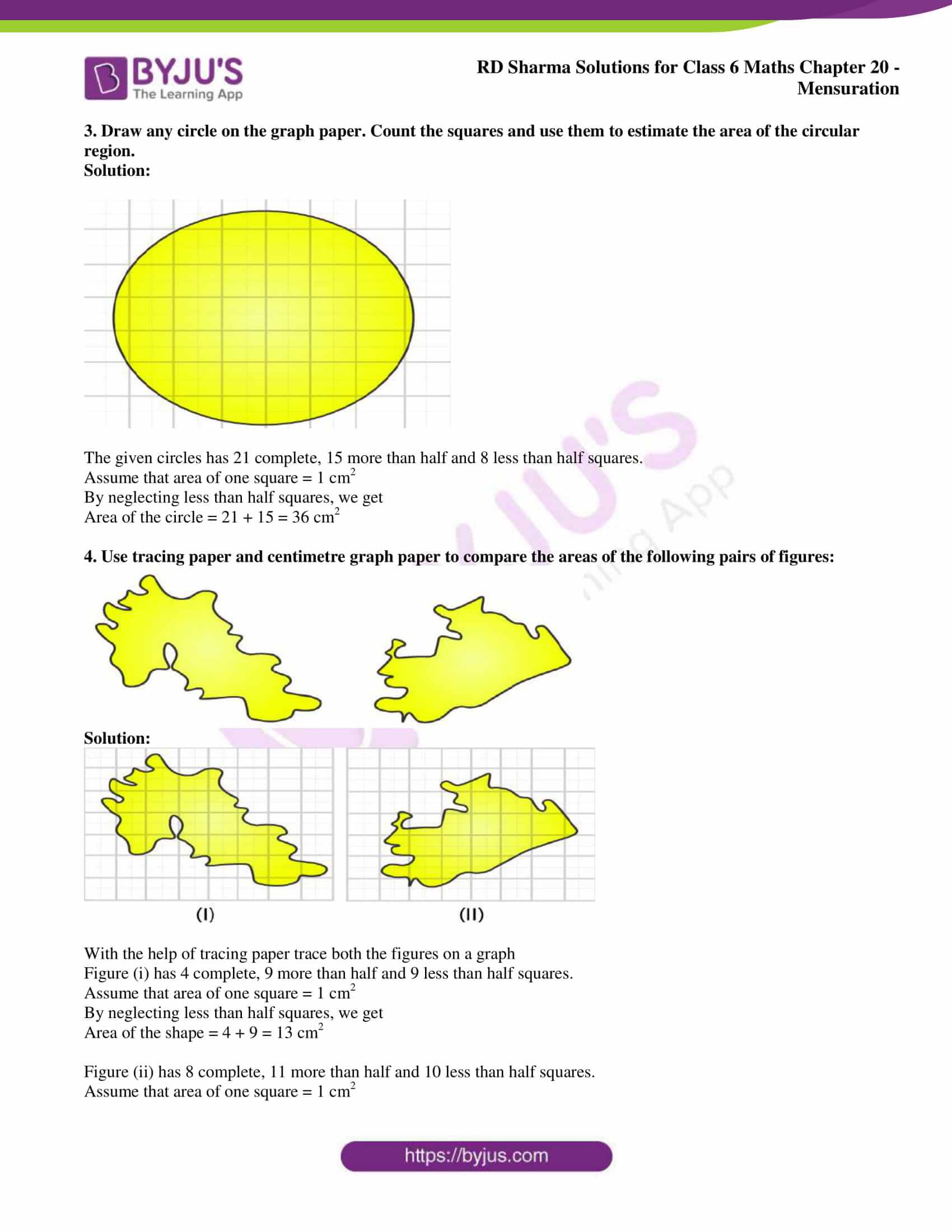### Access answers to Maths RD Sharma Solutions for Class 6 Chapter 20: Mensuration Exercise 20.3

1. The following figures are drawn on a squared paper. Count the number of squares enclosed by each figure and find its area, taking the area of each square as 1 cm2. (Fig. 20.25).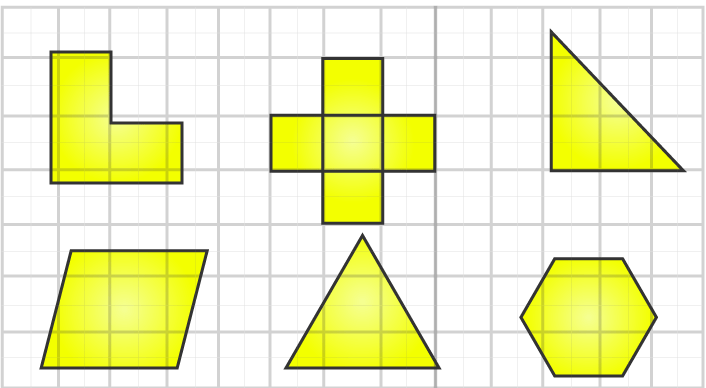Solution:

(i) The given shape has 16 complete squares.

It is given that area of one square = 1 cm2

So the area of the given shape = 16 × 1 = 16 cm2

(ii) The given shape has 36 complete squares.

It is given that area of one square = 1 cm2

So the area of the given shape = 36 × 1 = 36 cm2

(iii) The given shape has 15 complete and 6 half squares.

It is given that area of one square = 1 cm2

So the area of the given shape = 15 + 6 × 12 = 18 cm2

(iv) The given shape has 20 complete and 8 half squares.

It is given that area of one square = 1 cm2

So the area of the given shape = 20 + 8 × 12 = 24 cm2

(v) The given shape has 13 complete, 8 more than half and 7 less than half squares.

It is given that area of one square = 1 cm2

So the area of the given shape = 13 + 8 × 1 = 21 cm2

(vi) The given shape has 8 complete, 6 more than half and 4 less than half squares.

It is given that area of one square = 1 cm2

So the area of the given shape = 8 + 6 × 1 = 14 cm2

2. On a squared paper, draw (i) a rectangle, (ii) a triangle (iii) any irregular closed figure. Find the approximate area of each by counting the number of squares complete, more than half and exactly half.

Solution:

(i) A rectangle

The given shape has 18 complete squares

Assume that area of one square = 1 cm2

So the area of the rectangle = 18 × 1 = 18 cm2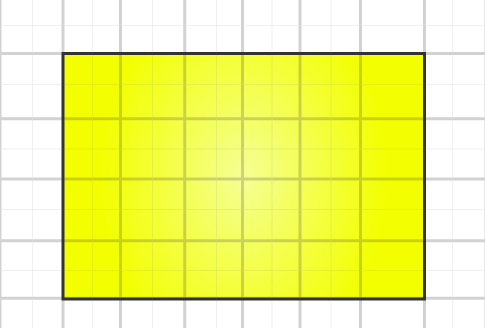(ii) A triangle

The given shape has 4 complete, 6 more than half and 6 less than half squares.

Assume that area of one square = 1 cm2

So the area of the square = 4 + 6 × 1 = 10 cm2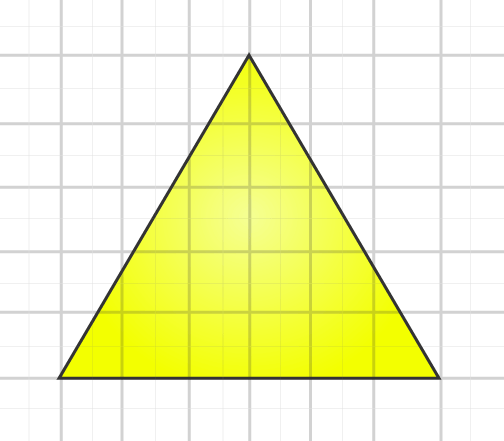(iii) Any irregular figure

The given shape has 10 complete, 1 exactly half, 7 more than half and 6 less than half squares.

Assume that area of one square = 1 cm2

So the area of the shape = 10 + 1 × 12 + 7 × 1 = 17.5 cm2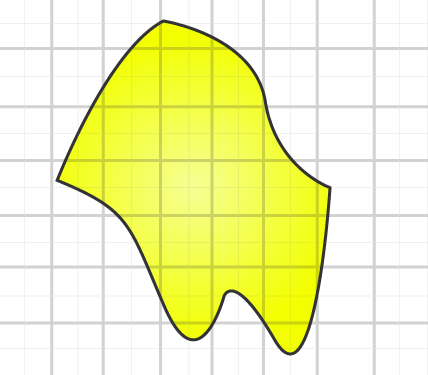3. Draw any circle on the graph paper. Count the squares and use them to estimate the area of the circular region.

Solution: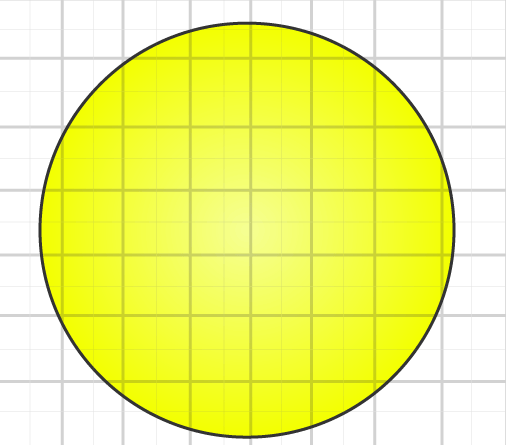The given circles has 21 complete, 15 more than half and 8 less than half squares.

Assume that area of one square = 1 cm2

By neglecting less than half squares, we get

Area of the circle = 21 + 15 = 36 cm2

4. Use tracing paper and centimetre graph paper to compare the areas of the following pairs of figures: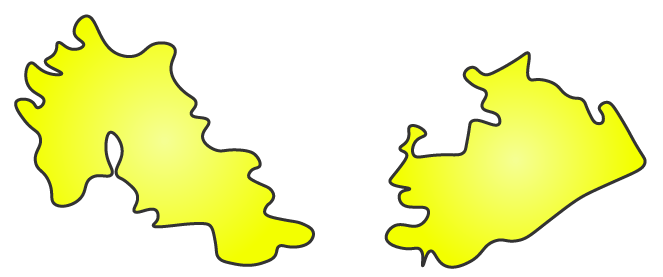Solution: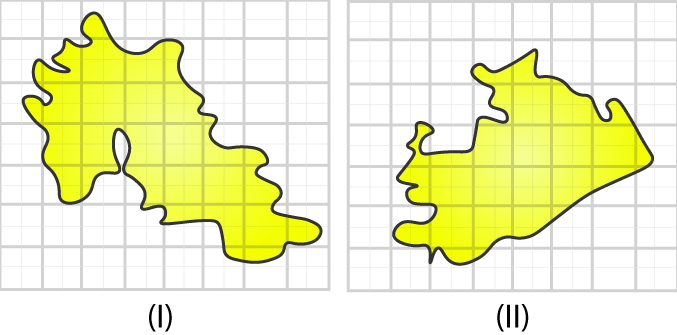With the help of tracing paper trace both the figures on a graph

Figure (i) has 4 complete, 9 more than half and 9 less than half squares.

Assume that area of one square = 1 cm2

By neglecting less than half squares, we get

Area of the shape = 4 + 9 = 13 cm2

Figure (ii) has 8 complete, 11 more than half and 10 less than half squares.

Assume that area of one square = 1 cm2

By neglecting less than half squares, we get

Area of the shape = 8 + 11 = 19 cm2

By comparing the areas of both the shapes, we know that the figure (ii) has area greater than that of figure (i).